Potential difference between the points P and Q in the electric circuit shown is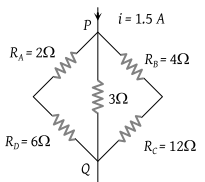(1) 4.5 V

(2) 1.2 V

(3) 2.4 V

(4) 2.88 V

Concept Questions :-

Combination of resistors
High Yielding Test Series + Question Bank - NEET 2020

Difficulty Level:

The current between B and D in the given figure is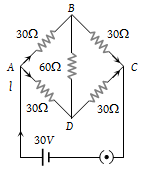(1) 1 amp

(2) 2 amp

(3) Zero

(4) 0.5 amp

Concept Questions :-

Combination of resistors
High Yielding Test Series + Question Bank - NEET 2020

Difficulty Level:

In the given figure, equivalent resistance between A and B will be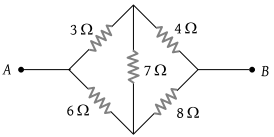(1) $\frac{14}{3}\text{\hspace{0.17em}\hspace{0.17em}}\Omega$

(2) $\frac{3}{14}\text{\hspace{0.17em}\hspace{0.17em}}\Omega$

(3) $\frac{9}{14}\text{\hspace{0.17em}\hspace{0.17em}}\Omega$

(4) $\frac{14}{9}\text{\hspace{0.17em}\hspace{0.17em}}\Omega$

Concept Questions :-

Combination of resistors
High Yielding Test Series + Question Bank - NEET 2020

Difficulty Level:

In a typical Wheatstone network, the resistances in cyclic order are A = 10 Ω, B = 5 Ω, C = 4 Ω and D = 4 Ω for the bridge to be balanced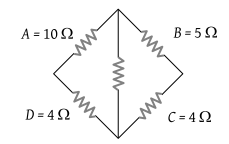(1) 10 Ω should be connected in parallel with A

(2) 10 Ω should be connected in series with A

(3) 5 Ω should be connected in series with B

(4) 5 Ω should be connected in parallel with B

Concept Questions :-

Combination of resistors
High Yielding Test Series + Question Bank - NEET 2020

Difficulty Level:

In the circuit shown in figure, the current drawn from the battery is 4A. If 10 Ω resistor is replaced by 20 Ω resistor, then current drawn from the circuit will be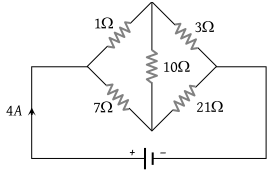(1) 1 A

(2) 2 A

(3) 3 A

(4) 0 A

Concept Questions :-

Combination of resistors
High Yielding Test Series + Question Bank - NEET 2020

Difficulty Level:

The equivalent resistance between P and Q in the given figure, is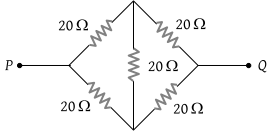(1) 50 Ω

(2) 40 Ω

(3) 30 Ω

(4) 20 Ω

Concept Questions :-

Combination of resistors
High Yielding Test Series + Question Bank - NEET 2020

Difficulty Level:

If each of the resistance of the network shown in the figure is R, the equivalent resistance between A and B is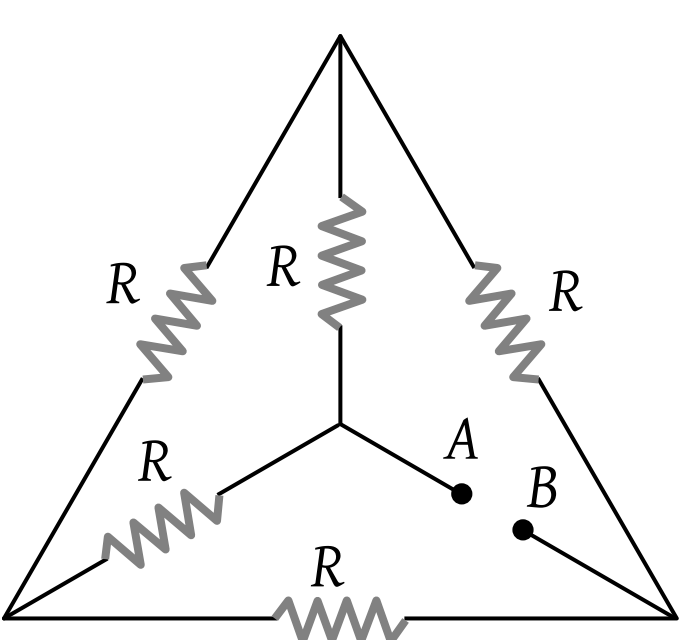(1) 5 R

(2) 3 R

(3) R

(4) R/2

Concept Questions :-

Combination of resistors
High Yielding Test Series + Question Bank - NEET 2020

Difficulty Level:

The equivalent resistance of the following diagram A and B is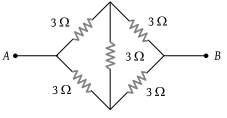(1) $\frac{2}{3}\Omega$

(2) 9 Ω

(3) 6 Ω

(4) None of these

Concept Questions :-

Combination of resistors
High Yielding Test Series + Question Bank - NEET 2020

Difficulty Level:

Thirteen resistances each of resistance R ohm are connected in the circuit as shown in the figure below. The effective resistance between A and B is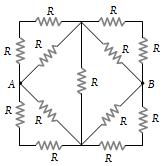(1) 2R Ω

(2) $\frac{4R}{3}\text{\hspace{0.17em}\hspace{0.17em}}\Omega$

(3) $\frac{2\text{\hspace{0.17em}}R}{3}\text{\hspace{0.17em}\hspace{0.17em}}\Omega$

(4) R Ω

Concept Questions :-

Combination of resistors
High Yielding Test Series + Question Bank - NEET 2020

Difficulty Level:

For what value of unknown resistance X, the potential difference between B and D will be zero in the circuit shown in the figure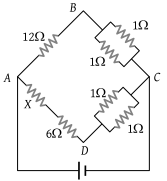(1) 4 Ω

(2) 6 Ω

(3) 2 Ω

(4) 5 Ω

Concept Questions :-

Combination of resistors